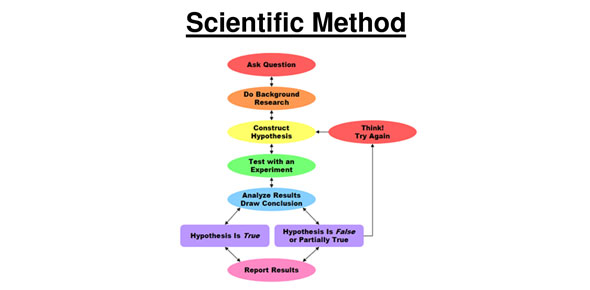# Quiz Questions Over Scientific Method

12 Questions | Total Attempts: 1203SettingsPlease put first and last name and your class period in the "Name" box. For example: Carla Williams 2R

• 1.
Science starts with a
• A.

Question

• B.

• C.

S

• 2.
The second step of the scientific method is
• A.

• B.

Form a hypothesis

• C.

Design and conduct an experiment

• 3.
The third step of the scientific method is
• A.

Form a hypothesis

• B.

Analyze results

• C.

Design and conduct an experiment

• D.

Communicate or share results

• 4.
The fourth step of the scientific method is
• A.

Analyze results

• B.

Form a hypothesis

• C.

• D.

Design and conduct an experiment

• E.

Draw conclusions

• 5.
The fifth step of the scientific method is
• A.

Draw conclusion

• B.

Analyze results

• C.

Design and conduct an experiment

• D.

Communicate or share results

• 6.
How many steps are there to the scientific method?
• A.

4

• B.

5

• C.

6

• 7.
The last step of the scientific method is
• A.

Draw conclusion

• B.

Analyze results

• C.

Design and conduct an experiment

• D.

Communicate or share results

• 8.
You should conduct an experiment before you make a hypothesis.
• A.

True

• B.

False

• 9.
Ask a question and form a hypothesis are part of which stage of the scientific method?
• A.

Pre-experimental stage

• B.

Experimental stage

• C.

Post-experimental stage

• 10.
Which of the following are part of the experimental stage?
• A.

Gathering materials

• B.

Know how to use the equipment

• C.

Observe and record data

• D.

Identify a single test variable and control the other variables

• E.

Perform measurements

• F.

Use mathematics

• G.

Form a hypothesis

• H.

• 11.
Which step(s) are part of the post-experimental stage?
• A.

Analyze the results of the experiment

• B.

Draw a conclusion

• C.

Communicate or share results

• D.

Analyze the results of the experiment and draw a conclusion

• E.

Draw a conclusion and communicate results

• F.

Analyze the results of the experiment, draw a conclusion, and communicate the results

• 12.
The first step of the scientific method is
• A.

Form a hypothesis

• B.Back to top